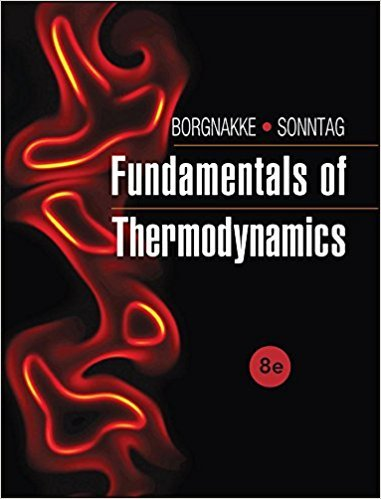×
Log in to StudySoup
Get Full Access to Fundamentals Of Thermodynamcs - 8 Edition - Chapter 3 - Problem 140hp
Join StudySoup for FREE
Get Full Access to Fundamentals Of Thermodynamcs - 8 Edition - Chapter 3 - Problem 140hp

Already have an account? Login here
×
Reset your password

# A rigid container has 2 kg of carbon dioxide gas at 100ISBN: 9781118131992 58

## Solution for problem 140HP Chapter 3

Fundamentals of Thermodynamcs | 8th Edition

• Textbook Solutions
• 2901 Step-by-step solutions solved by professors and subject experts
• Get 24/7 help from StudySoup virtual teaching assistantsFundamentals of Thermodynamcs | 8th Edition

4 5 1 372 Reviews
20
0
Problem 140HP

A rigid container has 2 kg of carbon dioxide gas at 100 kPa and 1200 K that is heated to 1400 K. Solve for the heat transfer using (a) the heat capacity from Table A.5 and (b) properties from Table A.8.

Step-by-Step Solution:

Solution 140 HP

Step 1 of 5

For a rigid container of carbon dioxide gas,  we need to find the heat transfer using (a) the heat capacity from given Table A.5, and (b) the properties from Table A.8

Step 2 of 5

Step 3 of 5

##### ISBN: 9781118131992

The full step-by-step solution to problem: 140HP from chapter: 3 was answered by , our top Engineering and Tech solution expert on 08/03/17, 05:05AM. The answer to “?A rigid container has 2 kg of carbon dioxide gas at 100 kPa and 1200 K that is heated to 1400 K. Solve for the heat transfer using (a) the heat capacity from Table A.5 and (b) properties from Table A.8.” is broken down into a number of easy to follow steps, and 41 words. This textbook survival guide was created for the textbook: Fundamentals of Thermodynamcs , edition: 8. Fundamentals of Thermodynamcs was written by and is associated to the ISBN: 9781118131992. Since the solution to 140HP from 3 chapter was answered, more than 339 students have viewed the full step-by-step answer. This full solution covers the following key subjects: kmol, dioxide, Table, properties, gas. This expansive textbook survival guide covers 7 chapters, and 1462 solutions.

Unlock Textbook Solution

Enter your email below to unlock your verified solution to:

A rigid container has 2 kg of carbon dioxide gas at 100